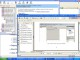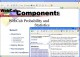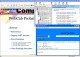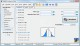WebCab Probability and Stat for Delphi 3.6 Statistics, Discrete Prob, Distributions, Hypo. testing, Correlation,Regression DemoWebCab Probability and Stat (J2EE Ed.) 3.6 Statistics, Discrete Prob, Distributions, Hypo. testing, Correlation,Regression DemoWebCab Probability and Stat (J2SE Ed.) 3.6 Statistics, Discrete Prob, Distributions, Hypo. testing, Correlation,Regression DemoWebCab Probability and Stat for .NET 3.6 Statistics, Discrete Prob, Distributions, Hypo. testing, Correlation,Regression DemoWebCab Probability and Stat for .NET 3.3 Statistics, Discrete Prob, Distributions, Hypo. testing, Correlation,Regression DemoMathAid Probability and Statistics 25.63 Java based math course includes problem-solving lessons, drills and tests DemoWebCab Probability and Stat (J2SE Ed.) 3.3 Statistics, Discrete Prob, Distributions, Hypo. testing, Correlation,Regression DemoESBPDF Analysis - Probability Software 2.4.1 Probability Analysis Software for Windows that is easy to use. SharewareDomino Probability 1.0 Students are shown a set of dominos and asked the probability of a certain outcome, such as picking a domino whose two numbers sum to eight. FreewareProbability Calc 1.0 Probability Calc offers you an application that calculates the probability of z, t, F, and chi-square values. Freeware